# Numeration Systems

## Digital Circuits

• #### Question 1

Digital computers communicate with external devices through ports: sets of terminals usually arranged in groups of 4, 8, 16, or more (4 bits = 1 nybble, 8 bits = 1 byte, 16 bits = 2 bytes). These terminals may be set to high or low logic states by writing a program for the computer that sends a numerical value to the port. For example, here is an illustration of a micro controller being instructed to send the hexadecimal number F3 to port A and 2C to port B: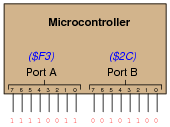Suppose we wished to use the upper four bits of port A (pins 7, 6, 5, and 4) to drive the coils of a stepper motor in this eight-step sequence:

Step 1:
0001
Step 2:
0011
Step 3:
0010
Step 4:
0110
Step 5:
0100
Step 6:
1100
Step 7:
1000
Step 8:
1001

As each pin goes high, it drives a power MOSFET on, which sends current through that respective coil of the stepper motor. By following a “shift” sequence as shown, the motor will rotate a small amount for each cycle.

Write the necessary sequence of numbers to be sent to port A to generate this specific order of bit shifts, in hexadecimal. Leave the lower four bit of port A all in the low logic state.

• #### Question 2

The numeration system we use in our daily lives is called base ten, also called decimal or denary. What, exactly, does “base ten” mean?

Given the following base-ten number, identify which digits occupy the “one’s place,” “ten’s place,” “hundred’s place,” and “thousand’s place,” respectively:

 5,183

• #### Question 3

Observe the following sequence of numbers:

00

01

02

03

04

05

06

07

08

09

10

11

12

13

14

15

16

17

18

19

20

21

.

.

.

What pattern(s) do you notice in the digits, as we count upward from 0 to 21 (and beyond)? This may seem like a very simplistic question (and it is!), but it is important to recognize any inherent patterns so that you may understand counting sequences in numeration systems with bases other than ten.

• #### Question 4

The ancient Mayans used a vigesimal, or base-twenty, numeration system in their mathematics. Each “digit” was a actually a composite of dots and/or lines, as such:To represent numbers larger than twenty, the Mayans combined multiple “digits” the same way we do to represent numbers larger than ten. For example:Based on the examples shown above, determine the place-weighting of each “digit” in the vigesimal numeration system. For example, in our denary, or base-ten, system, we have a one’s place, a ten’s place, a hundred’s place, and so on, each successive “place” having ten times the “weight” of the place before it. What are the values of the respective “places” in the Mayan system?

Also, determine the values of these Mayan numbers: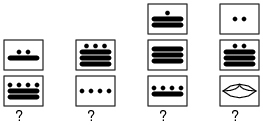• #### Question 5

Digital computers use a numeration system with a base of two, rather than a base of ten as we are accustomed to using. It is much easier to engineer circuitry that counts in “binary” than it is to design circuits that count in any other base system. Based on what you know of numeration systems, answer the following questions:

How many different symbols (ciphers) are there in the binary numeration system?
What are the different place-weight values in the binary system?
How would you represent the number “seventeen” in binary?
In our base-ten (denary) numeration system, each character is called a “digit.” What is each character called in the binary numeration system?
• #### Question 6

If a digital meter display has four digits, it can represent any number from 0000 to 9999. This constitutes ten thousand unique numbers representable by the display. How many unique numbers could be represented by five digits? By six digits?

If an ancient Mayan ledger had spaces for writing numbers with three “digits” each, how many unique numbers could be represented in each space? What if the spaces were expanded to hold four “digits” each?

If a digital circuit has four bits, how many unique binary numbers can it represent? If we expanded its capabilities to eight bits, how many unique numbers could be represented by the circuit?

After answering these questions, do you see any mathematical pattern relating the number of “places” in a numeration system and the number of unique quantities that may be represented, given the “base” value (“radix”) of the numeration system? Write a mathematical expression that solves for the number of unique quantities representable, given the “base” of the system and the number of “places”.

• #### Question 7

What is the largest number that can be counted to, in a base-ten system with six digits? How about in a base-twenty (vigesimal) system with four places? How about in a base-two (binary) system with ten bits?

• #### Question 8

How many binary bits are needed to count up to the number one million three hundred thousand seven hundred sixty two? Try to answer this question without converting this quantity into binary form, and then explain the mathematical procedure!

• #### Question 9

The circuit shown in this diagram is used to transmit a numerical value from one location to another, by means of switches and lights: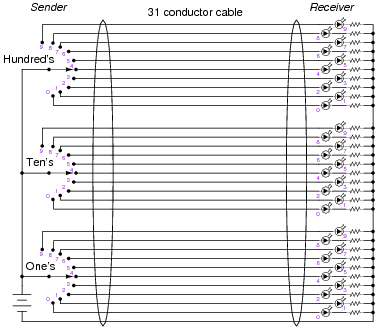Given the switches and lights shown, any whole number between 0 and 999 may be transmitted from the switch location to the light location.

In fact, the arrangement shown here is not too different from an obsolete design of electronic base-ten indicators known as Nixie tube displays, where each digit was represented by a neon-filled glass tube in which one of ten distinct electrodes (each in the shape of a digit, 0-9) could be energized, providing glowing numerals for a person to view.

However, the system shown in the above diagram is somewhat wasteful of wiring. If we were to use the same thirty-one conductor cable, we could represent a much broader range of numbers if each conductor represented a distinct binary bit, and we used binary rather than base-ten for the numeration system:How many unique numbers are representable in this simple communications system? Also, what is the greatest individual number which may be sent from the “Sender” location to the “Receiver” location?

• #### Question 10

Explain why binary is a natural numeration system for expressing numbers in electronic circuits. Why not decimal or some other base of numeration?

How do you suspect binary numbers may be stored in electronic systems, for future retrieval? What advantages are there to the use of binary numeration in storage systems?

• #### Question 11

Convert the following numbers from binary (base-two) to decimal (base-ten):

102 =
10102 =
100112 =
111002 =
101112 =
1010112 =
111001102 =
100011010112 =

Describe a general, step-by-step procedure for converting binary numbers into decimal numbers.

• #### Question 12

Convert the following numbers from decimal (base-ten) to binary (base-two):

710 =
1010 =
1910 =
25010 =
51110 =
82410 =
104410 =
924110 =

Describe a general, step-by-step procedure for converting decimal numbers into binary numbers.

• #### Question 13

A numeration system often used as a “shorthand” way of writing large binary numbers is the octal, or base-eight, system.

Based on what you know of place-weighted numeration systems, describe how many valid ciphers exist in the octal system, and the respective “weights” of each place in an octal number.

Also, perform the following conversions:

358 into decimal:
1610 into octal:
1100102 into octal:
518 into binary:
• #### Question 14

A numeration system often used as a “shorthand” way of writing large binary numbers is the hexadecimal, or base-sixteen, system.

Based on what you know of place-weighted numeration systems, describe how many valid ciphers exist in the hexadecimal system, and the respective “weights” of each place in a hexadecimal number.

Also, perform the following conversions:

3516 into decimal:
9316 into binary:
• #### Question 15

Complete this table, performing all necessary conversions between numeration systems:• #### Question 16

When representing non-whole numbers, we extend the “places” of our decimal numeration system past the right of the decimal point, like this: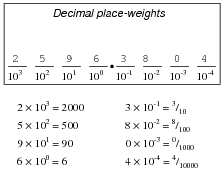How do you suppose we represent non-whole numbers in a numeration system with a base (or “radix”) other than ten? In the following examples, write the place-weight values underneath each place, and then determine the decimal equivalent of each example number: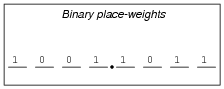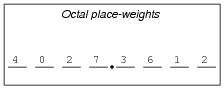• #### Question 17

Convert the following numbers (all between the values of 0 and 1) into decimal form:

0.0012 =
0.1012 =
0.101112 =
0.0058 =
0.3478 =
0.340718 =
0.00C16 =
0.A2F16 =
0.A2F0916 =
• #### Question 18

Complete this table, performing all necessary conversions between numeration systems. Truncate all answers to three characters past the point:• #### Question 19

In digital computer systems, binary numbers are often represented by a fixed number of bits, such as 8, or 16, or 32. Such bit groupings are often given special names, because they are so common in digital systems:

byte
nybble
word

How many binary bits is represented by each of the above terms?

And, for those looking for more challenge, try defining these terms:

nickle
deckle
chawmp
playte
dynner